Physics 357 : 1998 Lecture 21

Generations and Weak Isospin

The weak interaction couples the fermions in pairs, and the pairs are usually described as belonging to different generations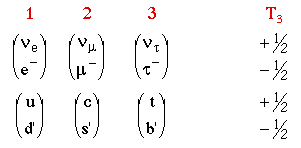The relative assignment of the quark and lepton generations is just according to their mass.

This two-state pairing is reminisent of the the two spin states allowed for a spin 1/2 fermion, or the 2 isospin states of a nucleon (proton and neutron), so we define all known fermions to have "weak isopin" 1/2, and the "up" type fermions are assigned T3=+1/2, and the "down" type fermions are assigned T3=-1/2.

Electroweak Theory

The group describing the spin states of a fermion is SU(2). So an SU(2) gauge theory is the "obvious" theory for the weak interaction. A gauge theory needs bosons to transform between all types of "charge" in the theory, i.e.

W+: T3=+1 {(T3=1/2) -> (T3=-1/2)}, e.g.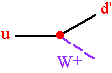W-: T3=-1 {(T3=-1/2) -> (T3=1/2)}, e.g.W0: T3=0 ·(+1/2-1/2)- (-1/2+1/2)Ò {(T31/2) -> (T31/2)}, e.g.But if this is true, then why is the rate for9 orders of magnitude smaller than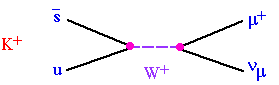?

Glashow, Iliopoulos, and Maiani showed (in 1970, before charm was discovered) that Cabibbo mixing along with the existence a charmed quark solves this problem. (See F&H equation 13.10.)

The W0 has the same quantum numbers as the photon, so mixing occurs leading to a unified electroweak theory.

Pure SU(2)L Bosons (Weak Isospin=1)

W+, W0, W-

Pure U(1) Boson (Weak Isospin=0)

B0

The actual observed bosons are the g and the Z0.

g = cosqW B0 - sinqW W0

Z0 = sinqW B0 + cosqW W0

Both the Z0 and the g are part SU(2) and part U(1) gauge bosons, so the coupling of the Z0 depends on both the electric charge and the T3 of the fermions. i.e. The coupling of the Z0 to "up" type quarks is different from the coupling to "down" type quarks, which is different from charged leptons, which is different from neutrinos.

Both the Z0 and the g couple only fermions to themselves.

e.g. Only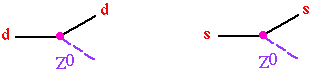Never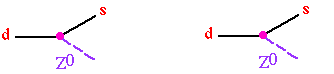Predictions of electroweak theory

• Neutral currents exist

e.g. (F&H equations 13.42, 13.43)Neutral currents discovered 1973 at CERN.

• W± and Z0 bosons exist, with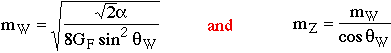(F&H equations 13.37, 13.38)

The W & Z were discovered in 1982 at CERN.

• the top quark must exist

or else the b quark will not have full weak coupling

The top quark was discovered in 1994 by the CDF experiment at Fermilab.

Some questions about electroweak gauge theory:

• Why is it left handed? SU(2)L!

WR?
• Why is gauge symmetry broken? MW,MZ>0! Masses?
• How is unitarity violation resolved? s(WW->WW) ~ s!

Higgs?